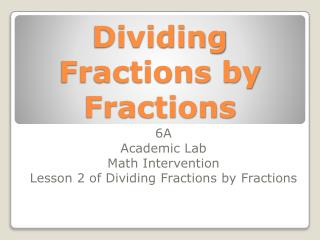DownloadDownload PresentationDividing Fractions by Fractions

# Dividing Fractions by Fractions

Télécharger la présentation## Dividing Fractions by Fractions

- - - - - - - - - - - - - - - - - - - - - - - - - - - E N D - - - - - - - - - - - - - - - - - - - - - - - - - - -
##### Presentation Transcript

1. Dividing Fractions by Fractions 6A Academic Lab Math Intervention Lesson 2 of Dividing Fractions by Fractions

2. Quick Review: Dividing Fractions by Whole Numbers Using Models • http://learnzillion.com/lessons/203-divide-fractions-by-whole-numbers-using-models • This video is just over 5 minutes. • Further Explanation: Dividing Fractions by Fractions Using Models • http://learnzillion.com/lessons/204-divide-fractions-by-fractions-using-models • This video is just 5 minutes. Let’s watch two video clips explaining what it means to divide fractions by fractions.

3. This array represents a pan of lasagna. • How many total pieces was the pan cut into? • 16 square pieces • How many pieces are remaining? • 10 square pieces • What fraction would represent the lasagna in this pan? • What fraction would represent the missing lasagna in this pan? • What fraction would represent a FULL pan of lasagna? What does it equal? Using arrays to model dividing fractions by fractions.

4. This array represents a pan of lasagna. If 4 people want to share the remaining lasagna, talk about how you could use the model to help to share it equally. Answers may vary. • One idea! You could assign a square for each person (P) and see how much lasagna each person will receive… TWO PIECES EACH!  • BUT…What do you do with the 2 squares that are leftover?  Remember, the people want to eat ALL of the remaining lasagna. Talk about how you could equally share the last two pieces of lasagna with four people. • One idea! You could divide the two square in HALF! • So how much lasagna will each person receive? 2 full square pieces and ½ of another which is the same as . Using arrays to model dividing fractions by fractions.

5. Using the same lasagna pan from the array model, let’s solve this division problem using the standard algorithm. To divide fractions by fractions, you MUST multiply the reciprocal. • Stay, Change, Changeand multiply across! • REDUCE to Lowest Terms! • can reduce to by cutting it in half and to reduces to in lowest terms! • If the fraction is improper rewrite it as a mixed number using basic division. • How many groups of 2, the denominator, are there in 5, the numerator? There are 2 full groups and half of another= 2! The Solution! Each person would receive 2 ½ pieces of the remaining lasagna in the pan. Using the standard algorithm to divide fractions by fractions

6. Array Model Solution Standard Algorithm: Calculation process/steps Each person would receive 2 full squares and ½ of another square for a total of 2½. To divide fractions by fractions, you MUST multiply the reciprocal. P2 P4 P1 P3 Compare the model to the algorithm, which method makes sense to you!

7. Brooke ate one slice of an apple pie, and of the pie was left over. She divided the rest of the apple pie equally among her 3 friends. What fraction of the whole pie did each person get? Student Practice- On a piece of paper, makean ARRAY MODEL to help you complete the division problem. Remember! Use a rectangle or square array!Pies and pizzas don’t have to be drawn as a circle!

8. Brooke ate one slice of an apple pie, and of the pie was left over. She divided the rest of the apple pie equally among her 3 friends. What fraction of the whole pie did each person get? First, create an array model with 10 equal size pieces then shade the remaining 9 pieces leaving the piece that Brooke ate empty. Second, equally share the remaining FULL pieces between the 3 friends. Third, count the number of pieces for each friend. Finally, how many equal pieces will each friend get? 3 out of 10 TOTAL pieces = 3/10 Student Practice- On a piece of paper, makean ARRAY MODEL to help you complete the division problem. Remember! Pies and pizzas don’t have to be drawn as a circle! Use a rectangle or square array!

9. Brooke ate one slice of an apple pie, and of the pie was left over. She divided the rest of the apple pie equally among her 3 friends. What fraction of the whole pie did each person get? Student Practice- On a piece of paper, solve this same problem but using the standard division of fractions algorithm.Remember! Use a rectangle or square array!Pies and pizzas don’t have to be drawn as a circle!

10. Brooke ate one slice of an apple pie, and of the pie was left over. She divided the rest of the apple pie equally among her 3 friends. What fraction of the whole pie did each person get? How much apple pie do we HAVE left or remaining? How many friends do we want to EQUALLY SHARE the pie with? Remember DIVIDING makes all the pieces the SAME size or EQUAL… otherwise it’s subtraction, if each friend gets a different size piece of pie. How do you write a whole number as a fraction? Put it over 1! Set up the problem in the order of the questions above! Stay, Change, Change and Multiply across! REDUCE to Lowest Terms, if needed!! IF the fraction is improper rewrite it as a mixed number using basic division. The Solution! Each person would receive 3 equal pieces of the remaining apple pie which is the same as 3/10 of the whole pie! 3 Student Practice- On a piece of paper, solve this same problem but using the standard division of fractions algorithm.